Next: Viscosity Up: Mathematical Models of Fluid Previous: Stress Tensor in a

# Stress Tensor in a Moving Fluid

We have seen that in a static fluid the stress tensor takes the form(1.15)

where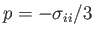is the static pressure: that is, minus the normal stress acting in any direction. The normal stress at a given point in a moving fluid generally varies with direction. In other words, the principal stresses are not equal to one another. However, we can still define the mean principal stress as. Moreover, given that the principal stresses are actually normal stresses (in a coordinate frame aligned with the principal axes), we can also regard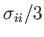as the mean normal stress. It is convenient to define pressure in a moving fluid as minus the mean normal stress: that is,(1.16)

Thus, we can write the stress tensor in a moving fluid as the sum of an isotropic part,, which has the same form as the stress tensor in a static fluid, and a remaining non-isotropic part,, which includes any shear stresses, and also has diagonal components whose sum is zero. In other words,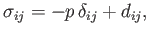(1.17)

where(1.18)

Moreover, because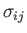and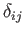are both symmetric tensors, it follows thatis also symmetric: that is,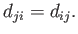(1.19)

It is clear that the so-called deviatoric stress tensor,, is a consequence of fluid motion, because it is zero in a static fluid. Suppose, however, that we were to view a static fluid both in its rest frame and in a frame of reference moving at some constant velocity relative to the rest frame. We would expect the force distribution within the fluid to be the same in both frames of reference, because the fluid does not accelerate in either. However, in the first frame, the fluid appears stationary and the deviatoric stress tensor is therefore zero, while in the second it has a spatially uniform velocity field and the deviatoric stress tensor is also zero (because it is the same as in the rest frame). We, thus, conclude that the deviatoric stress tensor is zero both in a stationary fluid and in a moving fluid possessing no spatial velocity gradients. This suggests that the deviatoric stress tensor is driven by velocity gradients within the fluid. Moreover, the tensor must vanish as these gradients vanish.

Let the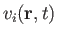be the Cartesian components of the fluid velocity at point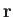and time. The various velocity gradients within the fluid then take the form. The simplest possible assumption, which is consistent with the previous discussion, is that the components of the deviatoric stress tensor are linear functions of these velocity gradients: that is,(1.20)

Here,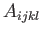is a fourth-order tensor (this follows from the quotient rule becauseandare both proper second-order tensors). Any fluid in which the deviatoric stress tensor takes the previous form is termed a Newtonian fluid, because Newton was the first to postulate a linear relationship between shear stresses and velocity gradients.

In an isotropic fluid--that is, a fluid in which there is no preferred direction--we would expect the fourth-order tensorto be isotropic--that is, to have a form in which all physical distinction between different directions is absent. As demonstrated in Section B.5, the most general expression for an isotropic fourth-order tensor is(1.21)

where,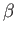, and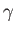are arbitrary scalars (which can be functions of position and time). Thus, it follows from Equations (1.20) and (1.21) that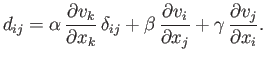(1.22)

However, according to Equation (1.19),is a symmetric tensor, which implies that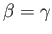, and(1.23)

where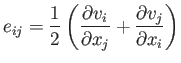(1.24)

is called the rate of strain tensor. Finally, according to Equation (1.18),is a traceless tensor, which yields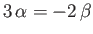, and(1.25)

where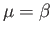. We, thus, conclude that the most general expression for the stress tensor in an isotropic Newtonian fluid is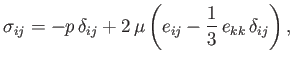(1.26)

where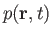andare arbitrary scalars.Next: Viscosity Up: Mathematical Models of Fluid Previous: Stress Tensor in a
Richard Fitzpatrick 2016-03-31